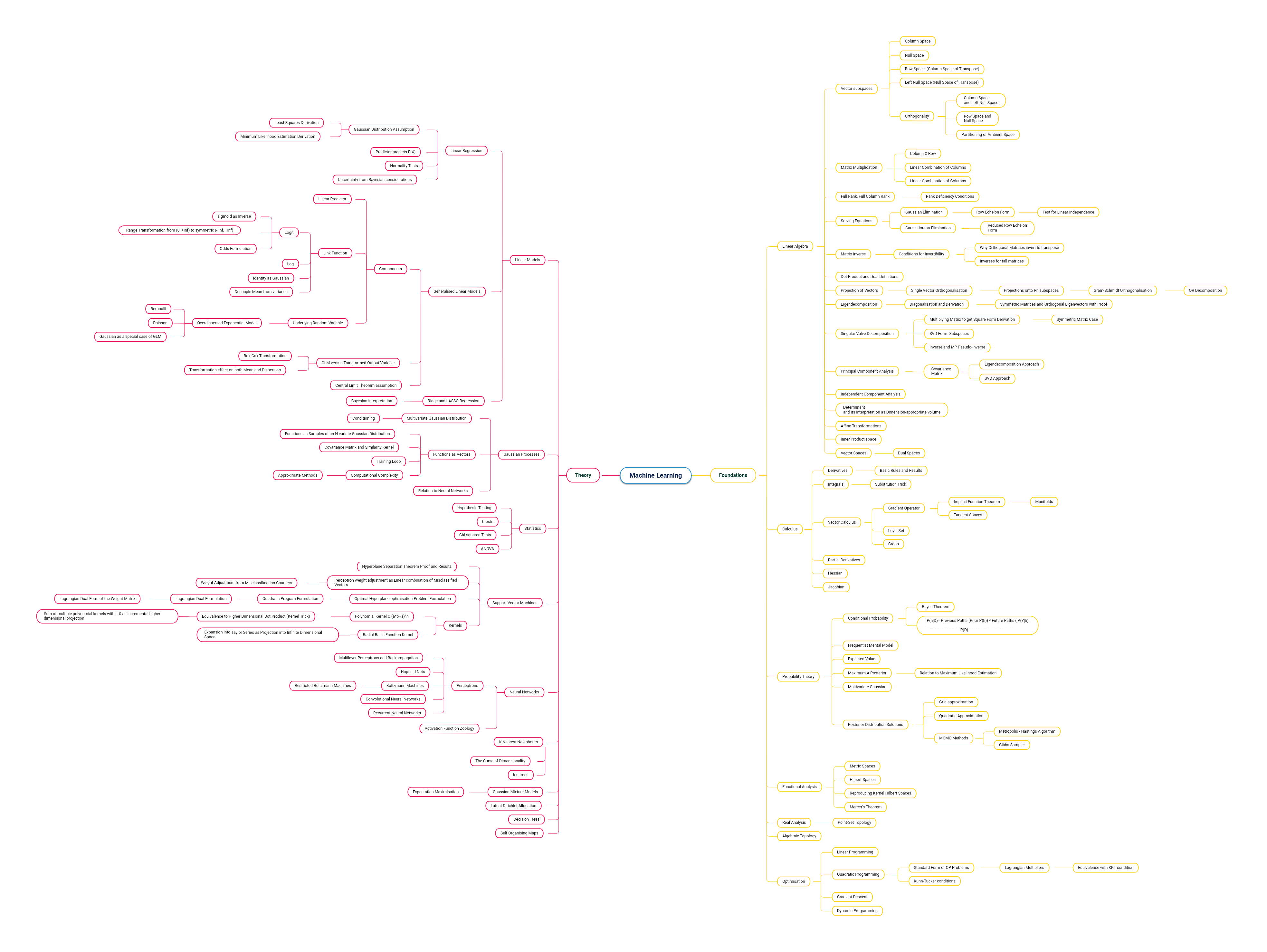# A Fish without a Bicycle

Technology and Art

## Machine Learning Theory Track

(Click the image below to see the full-size image)• ## Support Vector Machine from First Principles: Part One

Avishek Sen Gupta on 14 April 2021

We will derive the intuition behind Support Vector Machines from first principles. This will involve deriving some basic vector algebra proofs, including exploring some intuitions behind hyperplanes. Then we’ll continue adding to our understanding the concepts behind quadratic optimisation.

• ## Dot Product: Algebraic and Geometric Equivalence

Avishek Sen Gupta on 11 April 2021

The dot product of two vectors is geometrically simple: the product of the magnitudes of these vectors multiplied by the cosine of the angle between them. What is not immediately obvious is the algebraic interpretation of the dot product.

• ## Linear Regression: Assumptions and Results using the Maximum Likelihood Estimator

Avishek Sen Gupta on 5 April 2021

Let’s look at Linear Regression. The “linear” term refers to the fact that the output variable is a linear combination of the input variables.

• ## Matrix Rank and Some Results

Avishek Sen Gupta on 4 April 2021

I’d like to introduce some basic results about the rank of a matrix. Simply put, the rank of a matrix is the number of independent vectors in a matrix. Note that I didn’t say whether these are column vectors or row vectors; that’s because of the following section which will narrow down the specific cases (we will also prove that these numbers are equal for any matrix).

• ## Assorted Intuitions about Matrices

Avishek Sen Gupta on 3 April 2021

Some of these points about matrices are worth noting down, as aids to intuition. I might expand on some of these points into their own posts.

• ## Matrix Outer Product: Columns-into-Rows and the LU Factorisation

Avishek Sen Gupta on 2 April 2021

We will discuss the Column-into-Rows computation technique for matrix outer products. This will lead us to one of the important factorisations (the LU Decomposition) that is used computationally when solving systems of equations, or computing matrix inverses.

• ## Intuitions about the Orthogonality of Matrix Subspaces

Avishek Sen Gupta on 2 April 2021

This is the easiest way I’ve been able to explain to myself around the orthogonality of matrix spaces. The argument will essentially be based on the geometry of planes which extends naturally to hyperplanes.

• ## Matrix Outer Product: Value-wise computation and the Transposition Rule

Avishek Sen Gupta on 1 April 2021

We will discuss the value-wise computation technique for matrix outer products. This will lead us to a simple sketch of the proof of reversal of order for transposed outer products.

• ## Matrix Outer Product: Linear Combinations of Vectors

Avishek Sen Gupta on 30 March 2021

Matrix multiplication (outer product) is a fundamental operation in almost any Machine Learning proof, statement, or computation. Much insight may be gleaned by looking at different ways of looking matrix multiplication. In this post, we will look at one (and possibly the most important) interpretation: namely, the linear combination of vectors.

• ## Vectors, Normals, and Hyperplanes

Avishek Sen Gupta on 29 March 2021

Linear Algebra deals with matrices. But that is missing the point, because the more fundamental component of a matrix is what will allow us to build our intuition on this subject. This component is the vector, and in this post, I will introduce vectors, along with common notations of expression.

• ## Machine Learning Theory Track

Avishek Sen Gupta on 28 March 2021

I’ve always been fascinated by Machine Learning. This began in the seventh standard when I discovered a second-hand book on Neural Networks for my ZX Spectrum.

• ## First Post

Avishek Sen Gupta on 28 March 2021

My previous Wordpress site went kaput. With it went most of the content that I’ve posted over the years. I have switched over to Jekyll, and will be adding new material, as well as reintroducing old material as needed, from a backup that I have. Stay posted!